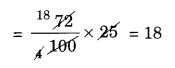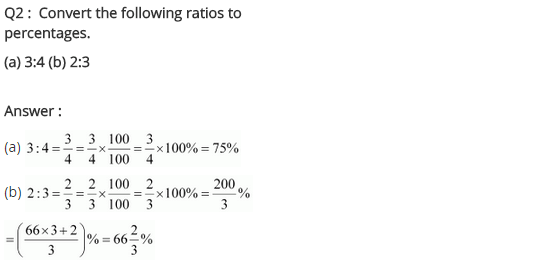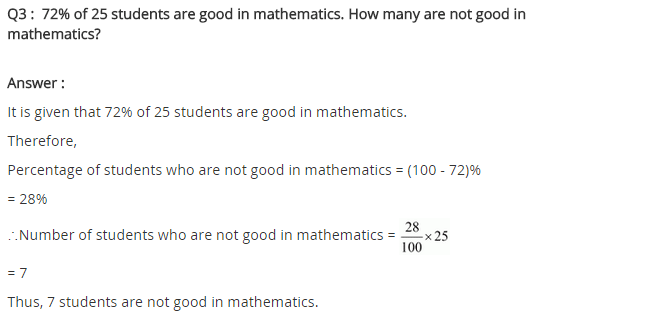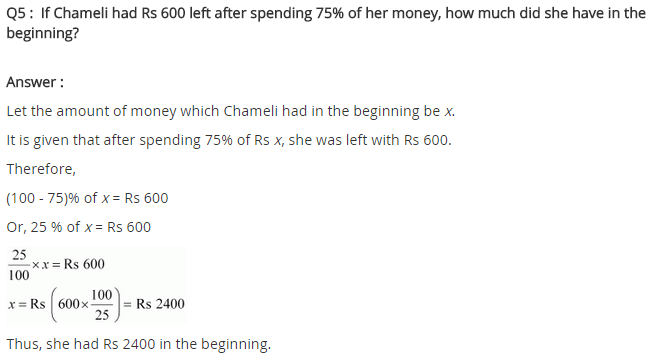## NCERT Solutions for Class 8 Maths Chapter 8 Comparing Quantities

NCERT Solutions for Class 8 Maths Chapter 8 Comparing Quantities Exercise 8.1

Ex 8.1 Class 8 Maths Question 1.
Find the ratio of the following:
(a) speed of a cycle 15 km per hour to the speed of scooter 30 km per hour.
(b) 5 m to 10 km
(c) 50 paise to ₹ 5
Solution:
(a) Speed of cycle : Speed of Scooter = 15 km per hour : 30 km per hour
= $$\frac { 15 }{ 30 }$$ = $$\frac { 1 }{ 2 }$$
Hence, the ratio = 1 : 2
(b) 5 m to 10 km
= 5 m : 10 × 1000 m [∵ 1 km = 1000 m]
= 5 m : 10000 m
= 1 : 2000
Hence, the ratio = 1 : 2000
(c) 50 paise to ₹ 5
= 50 paise : 5 × 100 paise
= 50 paise : 500 paise
ratio = 1 : 10

Ex 8.1 Class 8 Maths Question 2.
Convert the following ratios to percentages:
(a) 3 : 4
(b) 2 : 3
Solution:Ex 8.1 Class 8 Maths Question 3.
72% of 25 students are good in mathematics. How many are not good in mathematics?
Solution:
Number of students who are good in mathematics = 72% of 25Number of students who are not good in mathematics = 25 – 18 = 7

Ex 8.1 Class 8 Maths Question 4.
A football team won 10 matches out of the total number of matches they played. If their win percentage was 40, then how many matches did they play in all?
Solution:
40 matches the team won out of 100 matches
1 match was won out of $$\frac { 100 }{ 40 }$$ matches
10 matches the team will won out of $$\frac { 100 }{ 40 }$$ × 10 = 25 matches
Hence, the total number of matches played by the team = 25

Ex 8.1 Class 8 Maths Question 5.
If Chameli had ₹ 600 left after spending 75% of her money, how much did she have in the beginning?
Solution:
Let the money with Chameli be ₹ 100
Money spent by her = 75% of 100
= $$\frac { 75 }{ 100 }$$ × 100 = ₹ 75
The money left with her = ₹ 100 – ₹ 75 = ₹ 25
₹ 25 are left with her out of ₹ 100
₹ 1 is left with her out of ₹ $$\frac { 100 }{ 25 }$$
₹ 600 will be left out of ₹ $$\frac { 100 }{ 25 }$$ × 600 = ₹ 2400
Hence, she had ₹ 2400 in beginning.

Ex 8.1 Class 8 Maths Question 6.
If 60% of people in a city like a cricket, 30% like football and the remaining like other games, then what per cent of the people like other games? If the total number of people are 50 lakh, find the exact number who like each type of game.
Solution:
Total number of people = 50,00,000
Number of people who like cricket = 60% of 50,00,000
= $$\frac { 60 }{ 100 }$$ × 50,00,000
= 30,00,000
Number of people who like football = 30% of 50,00,000
= $$\frac { 30 }{ 100 }$$ × 50,00,000
= 15,00,000
Number of people who like other games = 50,00,000 – (30,00,000 + 15,00,000)
= 50,00,000 – 45,00,000
= 5,00,000
Percentage of the people who like other games = $$\frac { 500000 }{ 5000000 }$$ × 100 = 10%
Hence, 10% of people like other game.